1. The Einstein-DeSitter Model

The Einstein-DeSitter model is a matter dominated Friedmann model with zero curvature (k = 0). This model corresponds to a Minkowski universe (zero curvature), in which the universe will continue to expand forever with just the right amount of energy to escape to infinity. It is analogous to launching a rocket. If the rocket is given insufficient energy, it will be pulled back by the Earth. However, if its energy exceeds a certain critical velocity (escape velocity), it will continue into space with ever increasing speed. If it has exactly the escape velocity, it will proceed to escape the Earth with a velocity going to zero as the rocket approaches spatial infinity. The Einstein-DeSitter model corresponds to the universe having exactly the right escape velocity provided by the Big-Bang to escape the pull of gravity due to the matter in the universe.

By substituting k = 0 into (29) and (28), the Friedmann equations become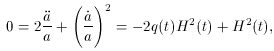(30)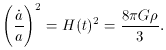(31)

By solving (31) for, a critical density can be found for a flat universe. The critical density is the amount of matter required for the universe to be exactly flat (k = 0) and is a function of time. The critical density at the present is defined as,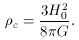(32)

If the density of the universe exceeds the critical density, the universe is open. Conversely, if the density is belowc the universe is open. For the observed Hubble parameter as defined in (2), the critical density today corresponds to a value,(33)

This is equivalent to roughly 10 hydrogen atoms per cubic meter. Although, this is incredibly small compared to Earthly standards, it must be remembered that most of space is empty and the concern is the total energy density.

Notice that the critical density depends on the Hubble constant. This means that the density required for a flat universe will change with time, in general, as the universe expands. For the universe to be `fine-tuned' to this precision is highly improbable; yet, most observations suggest this type of geometry. This paradoxical issue is referred to as the Flatness problem and will lead to one of the claimed triumphs of Inflation theory. Because it is believed that the universe is so close to being flat, it is useful to define the density parameter,.0 is the ratio of the density observed today,0, to the critical density,c. In general,is the ratio of the density to the critical value.

The quantitytogether with Equation (30), which implies q0 = 1/2, can be used to discriminate between the possible geometries for the matter dominated universe (see Table 2).

 Geometryq0 Fate of Universe Name Flat =1 1/2 Open Universe Einstein-DeSitter Model Hyperbolic <1 <1/2 Open Universe Open Model Spherical >1 >1/2 Closed Universe Closed

From the previous result for a matter dominated energy density, we found~ a-3. From this relation, conservation of energy follows,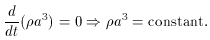This can be used to obtain a useful relation for,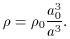Returning to the Friedmann equation (31) and substituting the above expression forone finds,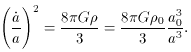Combining terms in a,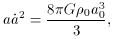now integrating,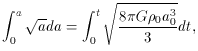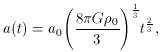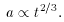(34)

So for the Einstein-DeSitter Model, the scale factor evolves as t2/3.